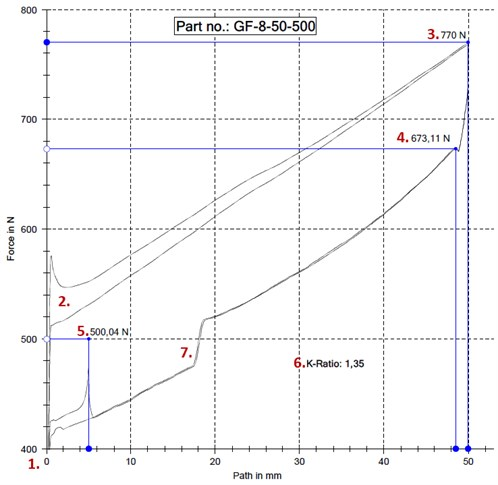# What is the force ratio of a gas spring?

The force quotient is a calculated value that indicates the force increase/loss between 2 measurement points.

The force in a compression gas spring increases the more it is compressed, in other words as the piston rod is pushed into the cylinder. This is because the gas in the cylinder is compressed more and more due to displacement changes inside the cylinder, thereby increasing the pressure that results in the axial force that pushes the piston rod.1. Force at unloaded length.When the spring is unloaded, it provides no force.
2. Force at initiation. Due to a combination of frictional force added to X number of N produced by the pressure in the cylinder, the curve clearly shows that the force rises pretty much as soon as a gas spring is compressed. Once the friction has been overcome the curve falls. If the spring has been at rest for some time, it may again require extra force to activate the gas spring. The example below shows the difference between the first and the second times the gas spring is compressed. If the gas spring is used regularly, the force curve will be close to the bottom curve. A gas spring that is at rest for some time will be more likely to be closer to the top curve.
3. Maximum force on compression.This force cannot really be used in structural contexts. The force is achieved only as a snapshot when the continuous pressure/travel stops. As soon as a gas spring is no longer travelling, the gas spring will attempt to return to its start position and therefore the usable force is less and the curve falls to point 4.
4. Maximum force yielded by a spring. This force is measured at the start of the gas spring’s recoil. This shows the correct image of how much maximum force a gas spring yields when it is stationary at this point.
5. Force provided by the gas spring in tables.By normal standards, the strength of the gas spring is provided from a measurement of the force at the remaining 5 mm travel towards its extended status, and at still status.
6. Force quotient.The force quotient is a calculated value that indicates the force increase/loss between values at point 5 and point 4. Thus a factor for how much force a gas spring loses on return from its maximum travel point 4, to point 5 (max. travel extended – 5 mm). The force quotient is calculated by dividing the force at point 4 by the value at point 5. This factor is also used in the reverse situation. If you have the force quotient (see value in our tables) and the force at point 5 (the force in our tables), the force at point 4 can be calculated by multiplying the force quotient by the force at point 5.
The force quotient is dependent on the volume in the cylinder combined with the thickness of the piston rod and the quantity of oil. This varies from size to size. Metals and fluids cannot be compressed, and it is therefore only the gas that can be compressed inside the cylinder.
7. Damping.Between point 4 and point 5 a bend can be seen in the force curve. It is at this point that the damping starts, and there is damping for the remaining part of the travel. Damping occurs through oil needing to seep through holes in the piston. By changing the combination of hole sizes, the quantity of oil, and oil viscosity, the damping can be changed.
Damping can/should not be removed entirely, as a fully compressed gas spring on sudden free movement of the piston will not be damped, and thereby the piston rod can be extended from the cylinder.

## Internet Explorer Incompatibility

This website unfortunately does not work well with Internet Explorer. We recommend using one of the following free browsers: